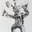Question-and-Answer Resource for the Building Energy Modeling Community
Get started with the Help pagehi all,

when looking at the heat and cooling zone summaries in energy plus results, there is one column for calculated design load and one column for user design load. Can anyone kindly explain what is the difference between the above mentioned loads?

edit retag close merge delete

Sort by » oldest newest most votedFrom the Output Details and Example documentation, Zone Sizing Information (eio output file - same information goes to both the eio output and table output):

Field: Calc Des Load {W} The unaltered zone load resulting from the zone sizing calculations, using the design weather and schedules specified in the input.

Field: User Des Load {W} User Des Load is the load after user specified adjustments and is the load actually used for sizing components. Following the calculations (as in the previous field) various adjustments can be made based on user input - the most obvious is a sizing factor; but it could also be something like cooling min air flow per zone area or just a user input design flow rate.

Field: Calc Des Air Flow Rate {m3/s} This is the calculated design air flow rate in m3/s. The calculated design air flow rate is the "non-adjusted" air flow rate. It is the air flow rate sufficient to meet the zone loads from the design day simulation.

Field: User Des Air Flow Rate {m3/s} User Des Air Flow Rate is the flow rate after user specified adjustments and is the flow rate actually used for sizing components. Following the calculations (as in the previous field) various adjustments can be made based on user input - the most obvious is a sizing factor; but it could also be something like cooling min air flow per zone area or just a user input design flow rate.

more

The user design load is simply the calculated design load multiplied by your Sizing:Zone "Zone Heating Sizing Factor" or "Zone Cooling Sizing Factor"

more